nLab horizontal chord diagram

For discussion of standard round chord diagrams see at chord diagram.

Context

Graph theory

graph theory

graph

category of simple graphs

Extra structure

Knot theory

knot theory

Examples/classes:

Types

knot invariants

Related concepts:

category: knot theory

Contents

Idea

A horizontal chord diagram on $n$ strands is a finite undirected graph that is obtained from a trivalent graph with $n$ numbered embedded disjoint circles by cutting the circles open (to give the strands), such that the result has all edges not inside the circles (the chords) be vertically ordered (i.e. along the strands) and going between distinct strands.

Here is an example of a horizontal chord diagram on 5 strands: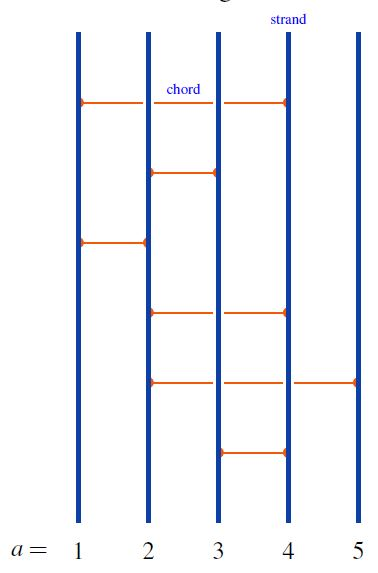graphics from Sati-Schreiber 19c

Defintions

Monoid of horizontal chord diagrams

For $n \in \mathbb{N}$ (the number of strands), the monoid of horizontal chord diagrams is the free monoid

(1)$\mathcal{D}^{pb}_n \;\coloneqq\; FreeMonoid \Big( \big\{ (i j) \,\vert\, 1 \leq i \lt j \leq n \big\} \Big)$

on the set of pairs of distinct elements of $\{1, \cdots, n\}$, i.e. of pairs of strands, called the chords (the traditional superscript $pb$ is for pure braids).

Hence a horizontal chord diagram is equivalently a finite list of chords

$D \;=\; (i_d j_d) \cdot \cdots \cdot (i_2 j_2) \cdot (i_1 j_1)$

and the product of chord diagrams is the concatenation of these list, with the empty list being the neutral element.

The function that sends the chord $(i j)$ to that permutation of $n$ elements (strands) which is given by the transposition $t_{i j}$ of the $i$th with the $j$th strand extends to a unique monoid homomorphism from the monoid of horizontal chord diagrams (1) to the symmetric group on $n$ elements:

$\array{ \mathcal{D}^{pn}_n &\overset{perm}{\longrightarrow}& Sym(n) \\ (i_d j_d) \cdot \cdots \cdot (i_1 j_1) &\mapsto& t_{i_d j_d} \circ \cdots \circ t_{i_1 j_1} \,. }$

Closure to round chord diagrams

Given a horizontal chord diagram on $n$ strands and given any choice of cyclic permutation of $n$ elements, the trace of horizontal to round chord diagrams is the round chord diagram obtained by gluing the ends of the strands according to the cyclic permutation, and retaining the chords in the evident way.

The following shows an example of the trace operation for cyclic permutation of strands one step to the left: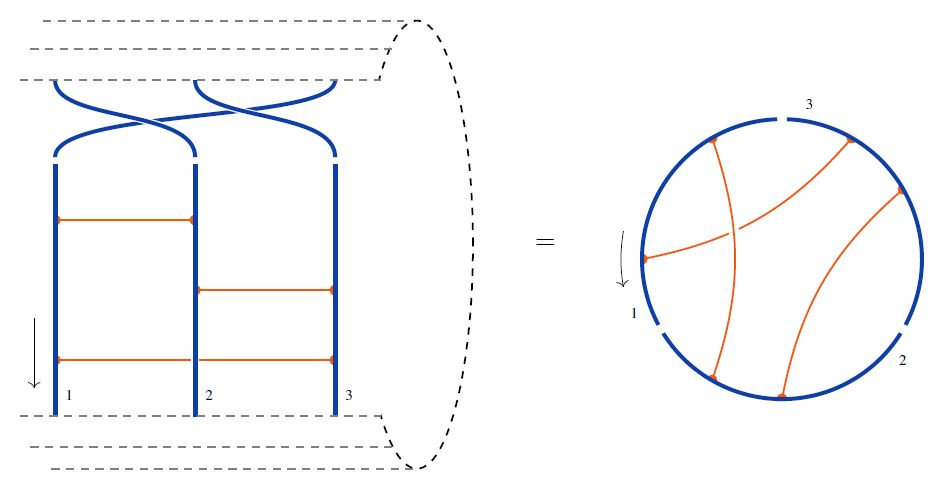graphics from Sati-Schreiber 19c

The following are the analogous traces of the four types of horizontal chord diagrams appearing in the 4T relation: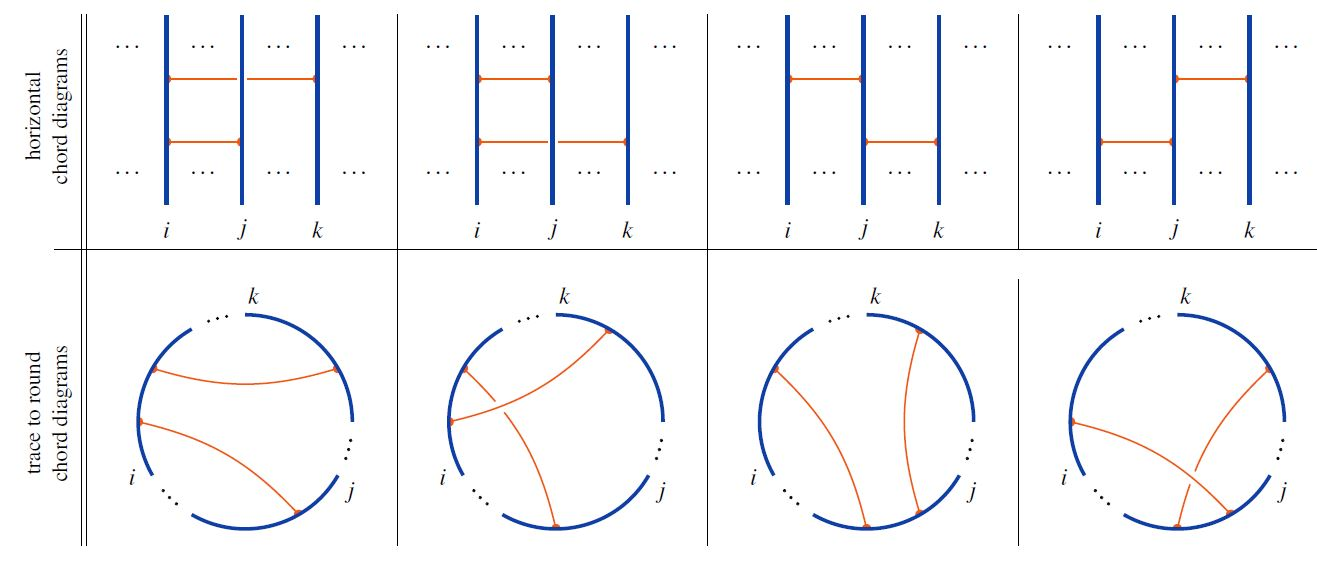graphics from Sati-Schreiber 19c

This defines a function

$tr \;\colon\; \mathcal{C}^{pb} \longrightarrow \mathcal{C}^c$

from the set of horizontal chord diagrams to the set of round chord diagrams.

Algebra of horizontal chord diagrams

Definition

For $n \in \mathbb{N}$ and for , the linear span $Span\big( \mathcal{D}_n^{pb}\big)$ on the set of horizontal chord diagrams on $n$ strands becomes an graded associative algebra

$\big( Span\big( \mathcal{D}_n^{pb}\big), \circ \big)$

under concatenation of strands.

For example: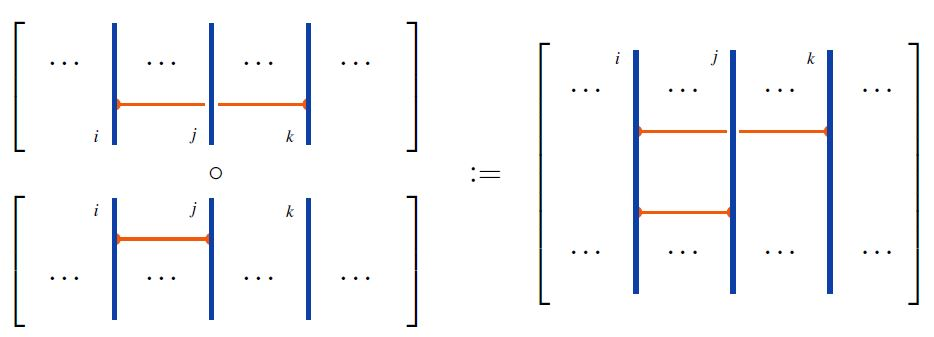The 2T- and 4T-relations

Definition

On the $R$-module $R\langle \mathcal{D}^{pb}\rangle$ of horizontal chord diagrams consider the following relations:

The 2T relations: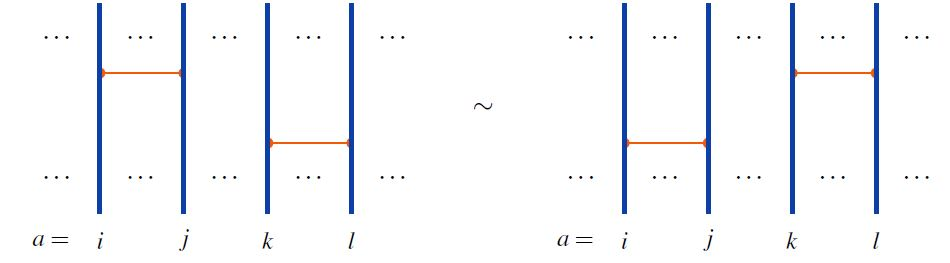and the 4T relations: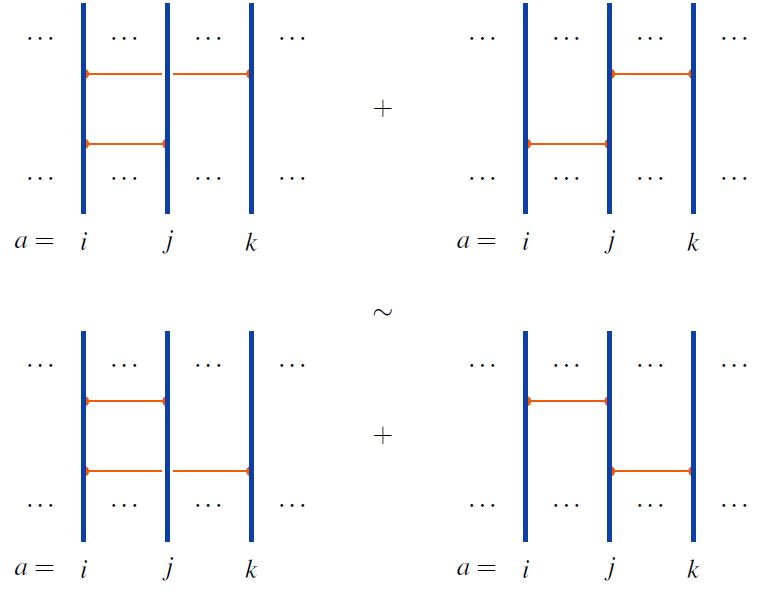graphics from Sati-Schreiber 19c

In terms of the commutator Lie algebra of the above algebra $\big( R\langle \mathcal{D}^{pb}\rangle, \circ \big)$ of horizontal chord diagrams, these are the infinitesimal braid relations.

One writes

(2)$\mathcal{A}^{{}^{pb}} \;\coloneqq\; R\langle \mathcal{D}^{pb}\rangle/(2T,4T)$

for the quotient algebra of horizontal chord diagrams by these relations.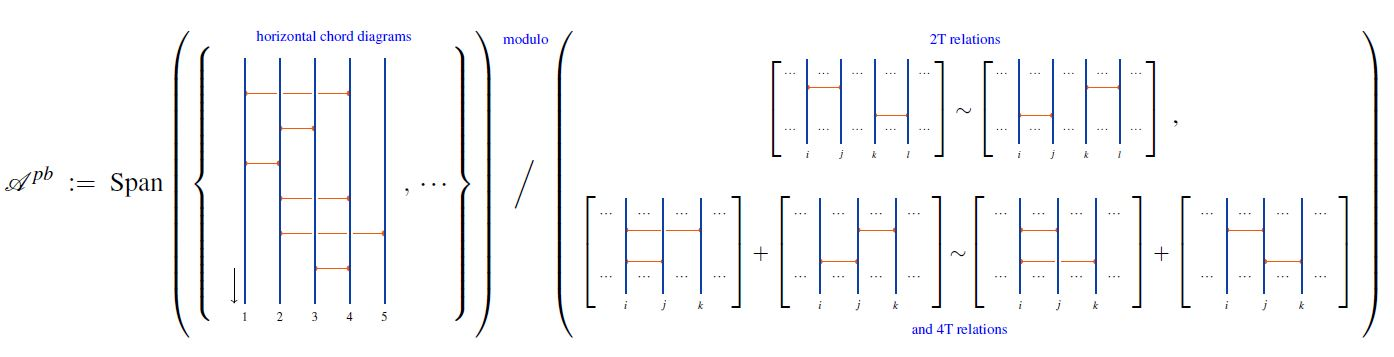Universal enveloping of infinitesimal braid relations

Proposition

(universal enveloping algebra of infinitesimal braid Lie algebra is horizontal chord diagrams modulo 2T&4T)

$\Big( \mathcal{A}_n^{{}^{pb}} \;\coloneqq\; Span \big( \mathcal{D}_n^{pb} \big)/(2T, 4T) , \circ \Big)$

of horizontal chord diagrams on $n$ strands with product given by concatenation of strands (Def. ) modulo the 2T relations and 4T relations (Def. ) is isomorphic to the universal enveloping algebra of the infinitesimal braid Lie algebra (this Def.):

$\big(\mathcal{A}_n^{pb}, \circ\big) \;\simeq\; \mathcal{U}(\mathcal{L}_n(D)) \,.$

Horizontal weight systems

An $R$-linear map from the quotient module (2) of horizontal chord diagrams to $R$

(3)$w \;\colon\; \mathcal{A}_n^{{}^{pb}} \longrightarrow R$

is called a weight system on horizontal chord diagrams (of $n$ strands), or maybe a horizontal weight systems.

Hence for $R = k$ a field, the vector space of all horizontal weight systems is the degreewise dual vector space

$\mathcal{W}_{pb} \;\coloneqq\; \big( \mathcal{A}^{pb} \big)^\ast$

Star-algebra structure

Over a ground ring $R$ that is itself equipped with the structure of a star-algebra $\mathbb{F} \overset{(-)^\ast}{\to} \mathbb{F}$ (such as the real numbers, trivially, or the complex numbers via complex conjugation), we have that also the associative algebra (2)

$\Big( \mathcal{A}_n^{{}^{pb}} \;\coloneqq\; Span \big( \mathcal{D}_n^{pb} \big)/(2T, 4T) , \circ \Big)$

of horizontal chord diagrams on $n$ strands with product given by concatenation of strands (Def. ) modulo the 2T relations and 4T relations (Def. )

becomes a star-algebra with star-operation

$(-)^\ast \;:\; \mathcal{A}_n^{{}^{pb}} \longrightarrow \mathcal{A}_n^{{}^{pb}}$

given by reversing the orientation of strands: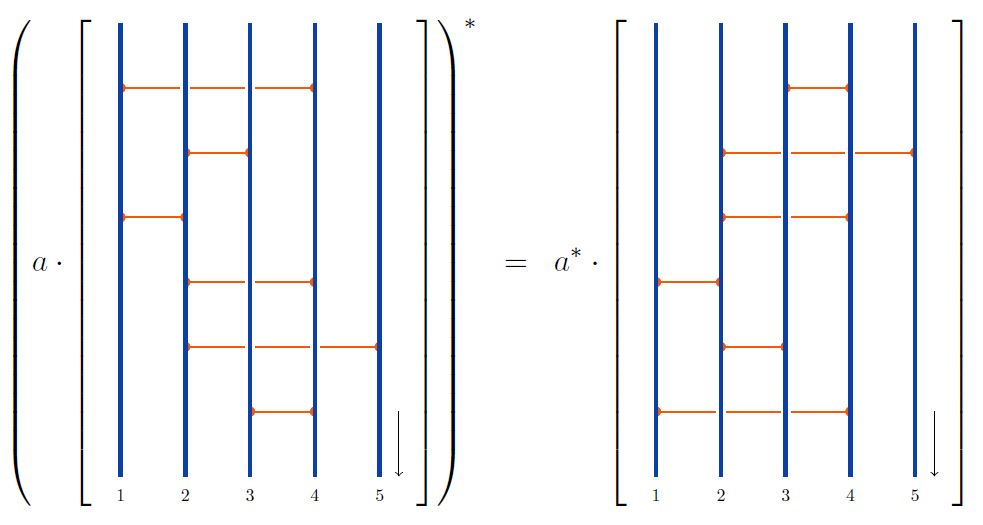Since horizontal chord diagrams are the homology of the loop space of configuration space and the homology of a loop space is an involutive Hopf algebra, this is a special case of the general fact that involutive Hopf algebras are star-algebras (here).

With respect to this star-algebra-structure one may ask (setting $R \coloneqq \mathbb{C}$ for definiteness) whether a given weight system (3)

$w \;\colon\; \mathcal{A}_n^{{}^{pb}} \longrightarrow \mathcal{C}$

is a state on a star-algebra in that for any $D \in \mathcal{A}_n^{{}^{pb}}$ we have that the value of $w$ on the corresponding normal operator $D \cdot D^\ast$ is a non-negative real number:

\Big( w \in \mathcal{W}_n^{{}^{pb}} \; \text{is a state} \Big) \phantom{AAA} \Leftrightarrow \phantom{AAA} \left( \begin{aligned} \text{1.}\;\;\; & w(1) = 1 \\ \text{2.}\;\;\; & \underset{ \mathclap{ D \in \mathcal{A}_n^{{}^{pb}} } }{ \forall } \;\;\;\; \Big( w(D \cdot D^\ast) \; \geq 0 \; \in \mathbb{R} \subset \mathbb{C} \Big) \end{aligned} \right) \,.

The weight systems which are states on a star-algebra with respect to this star-involution are discussed in CSS 21.

Closure to Sullivan chord diagrams

More generally, one obtains Sullivan chord diagrams with $p$ disjoint embedded circles from horizontal chord diagrams by closing up strands after acting with a permutation with $p$ cycles ($p$ orbits)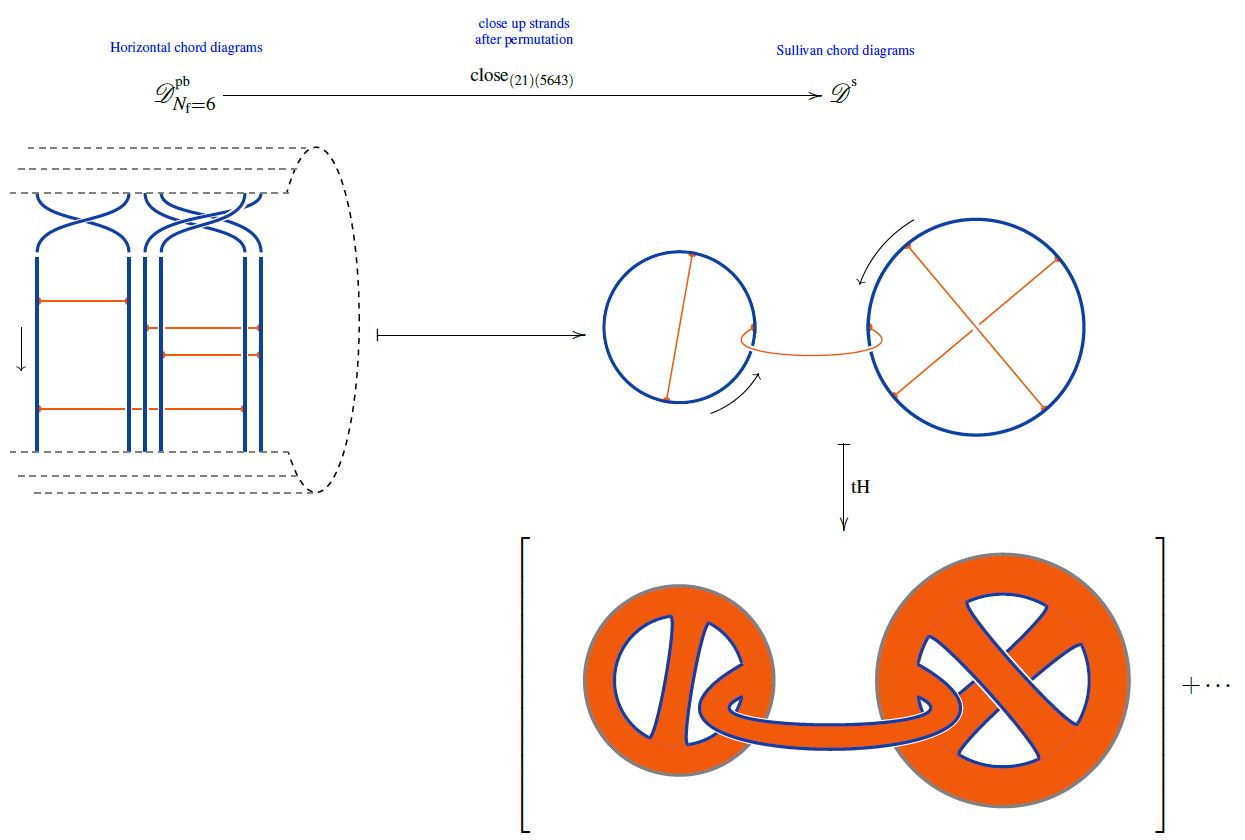Applications

Knizhnik-Zamolodchicov connection

For the Definition of the Knizhnik-Zamolodchikov connection we need the following notation:

1. configuration spaces of points

For $N_{\mathrm{f}} \in \mathbb{N}$ write

(4)$\underset{{}^{\{1,\cdots,N_{\mathrm{f}}\}}}{Conf}(\mathbb{R}^2) \;\coloneqq\; (\mathbb{R}^2)^n \backslash FatDiagonal$

for the ordered configuration space of n points in the plane, regarded as a smooth manifold.

Identifying the plane with the complex plane $\mathbb{C}$, we have canonical holomorphic coordinate functions

(5)$(z_1, \cdots, z_{N_{\mathrm{f}}}) \;\colon\; \underset{{}^{\{1,\cdots,n\}}}{Conf}(\mathbb{R}^2) \longrightarrow \mathbb{C}^{N_{\mathrm{f}}} \,.$
2. horizontal chord diagrams

(6)$\mathcal{A}^{{}^{pb}}_{N_{\mathrm{f}}} \;\coloneqq\; Span\big(\mathcal{D}^{{}^{pb}}_{N_{\mathrm{f}}}\big)/4T$

for the quotient vector space of the linear span of horizontal chord diagrams on $n$ strands by the 4T relations (infinitesimal braid relations), regarded as an associative algebra under concatenation of strands (here).

Definition

(Knizhnik-Zamolodchikov form)

(7)$\omega_{KZ} \;\in\; \Omega \big( \underset{{}^{\{1,\cdots,N_{\mathrm{f}}\}}}{Conf}(\mathbb{C}) \,, \mathcal{A}^{{}^{pb}}_{N_{\mathrm{f}}} \big)$

given in the canonical coordinates (5) by:

(8)$\omega_{KZ} \;\coloneqq\; \underset{ i \lt j \in \{1, \cdots, n\} }{\sum} d_{dR} log\big( z_i - z_j \big) \otimes t_{i j} \,,$

where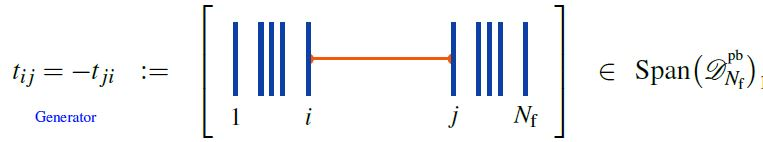is the horizontal chord diagram with exactly one chord, which stretches between the $i$th and the $j$th strand.

Regarded as a connection form for a connection on a vector bundle, this defines the universal Knizhnik-Zamolodchikov connection $\nabla_{KZ}$, with covariant derivative

$\nabla \phi \;\coloneqq\; d \phi + \omega_{KV} \wedge \phi$

for any smooth function

$\phi \;\colon\; \underset{{}^{\{1,\cdots,N_{\mathrm{f}}\}}}{Conf}(\mathbb{C}) \longrightarrow \mathcal{A}^{{}^{pb}}_{N_{\mathrm{f}}} Mod$

with values in modules over the algebra of horizontal chord diagrams modulo 4T relations.

The condition of covariant constancy

$\nabla_{KZ} \phi \;=\; 0$

is called the Knizhnik-Zamolodchikov equation.

Finally, given a metric Lie algebra $\mathfrak{g}$ and a tuple of Lie algebra representations

$( V_1, \cdots, V_{N_{\mathrm{f}}} ) \;\in\; (\mathfrak{g} Rep_{/\sim})^{N_{\mathrm{f}}} \,,$

the corresponding endomorphism-valued Lie algebra weight system

$w_{V} \;\colon\; \mathcal{A}^{{}^{pf}}_{N_{\mathrm{f}}} \longrightarrow End_{\mathfrak{g}}\big( V_1 \otimes \cdots V_{N_{\mathrm{f}}} \big)$

turns the universal Knizhnik-Zamolodchikov form (7) into a endomorphism ring-valued differential form

(9)$\omega_{KZ} \;\coloneqq\; \underset{ i \lt j \in \{1, \cdots, n\} }{\sum} d_{dR} log\big( z_i - z_j \big) \otimes w_V(t_{i j}) \;\in\; \Omega \big( \underset{{}^{\{1,\cdots,N_{\mathrm{f}}\}}}{Conf}(\mathbb{C}) \,, End\big(V_1 \otimes \cdot V_{N_{\mathrm{f}}} \big) \big) \,.$

The universal formulation (7) is highlighted for instance in Bat-Natan 95, Section 4.2, Lescop 00, p. 7. Most authors state the version after evaluation in a Lie algebra weight system, e.g. Kohno 14, Section 5.

Proposition

(Knizhnik-Zamolodchikov connection is flat)

The Knizhnik-Zamolodchikov connection $\omega_{ZK}$ (Def. ) is flat:

$d \omega_{ZK} + \omega_{ZK} \wedge \omega_{ZK} \;=\; 0 \,.$
Proposition

(Kontsevich integral for braids)

The Dyson formula for the holonomy of the Knizhnik-Zamolodchikov connection (Def. ) is called the Kontsevich integral on braids.

Chord diagrams as multi-trace observables in the BMN matrix model

The supersymmetric states of the BMN matrix model are temporally constant complex matrices which are complex metric Lie representations $\mathfrak{g} \otimes V \overset{\rho}{\to} V$ of $\mathfrak{g}=$su(2) (interpreted as fuzzy 2-sphere noncommutative geometries of giant gravitons or equivalently as fuzzy funnels of D0-D2 brane bound states).

A fuzzy 2-sphere-rotation invariant multi-trace observable on these supersymmetric states is hence an expression of the following form: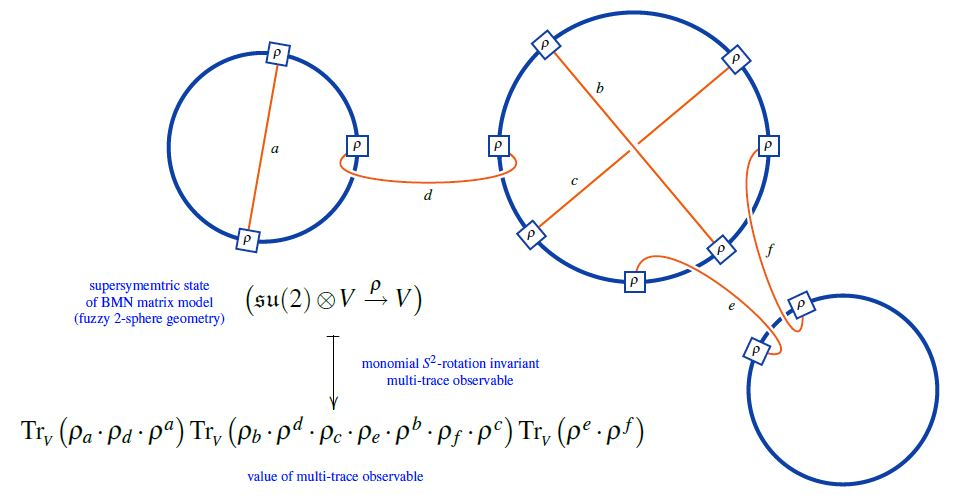(from Sati-Schreiber 19c)

Here we are showing the corresponding string diagram/Penrose notation for metric Lie representations, which makes manifest that

1. these multi-trace observables are encoded by Sullivan chord diagrams $D$

2. their value on the supersymmetric states $\mathfrak{su}(2) \otimes V \overset{\rho}{\to}V$ is the evaluation of the corresponding Lie algebra weight system $w_{{}_V}$ on $D$.

Or equivalently, if $\widehat D$ is a horizontal chord diagram whose $\sigma$-permuted closure is $D$ (see here) then the values of the invariant multi-trace observables on the supersymmetric states of the BMN matrix model are the evaluation of $w_{V,\sigma}$ on $\widehat D$, as shown here: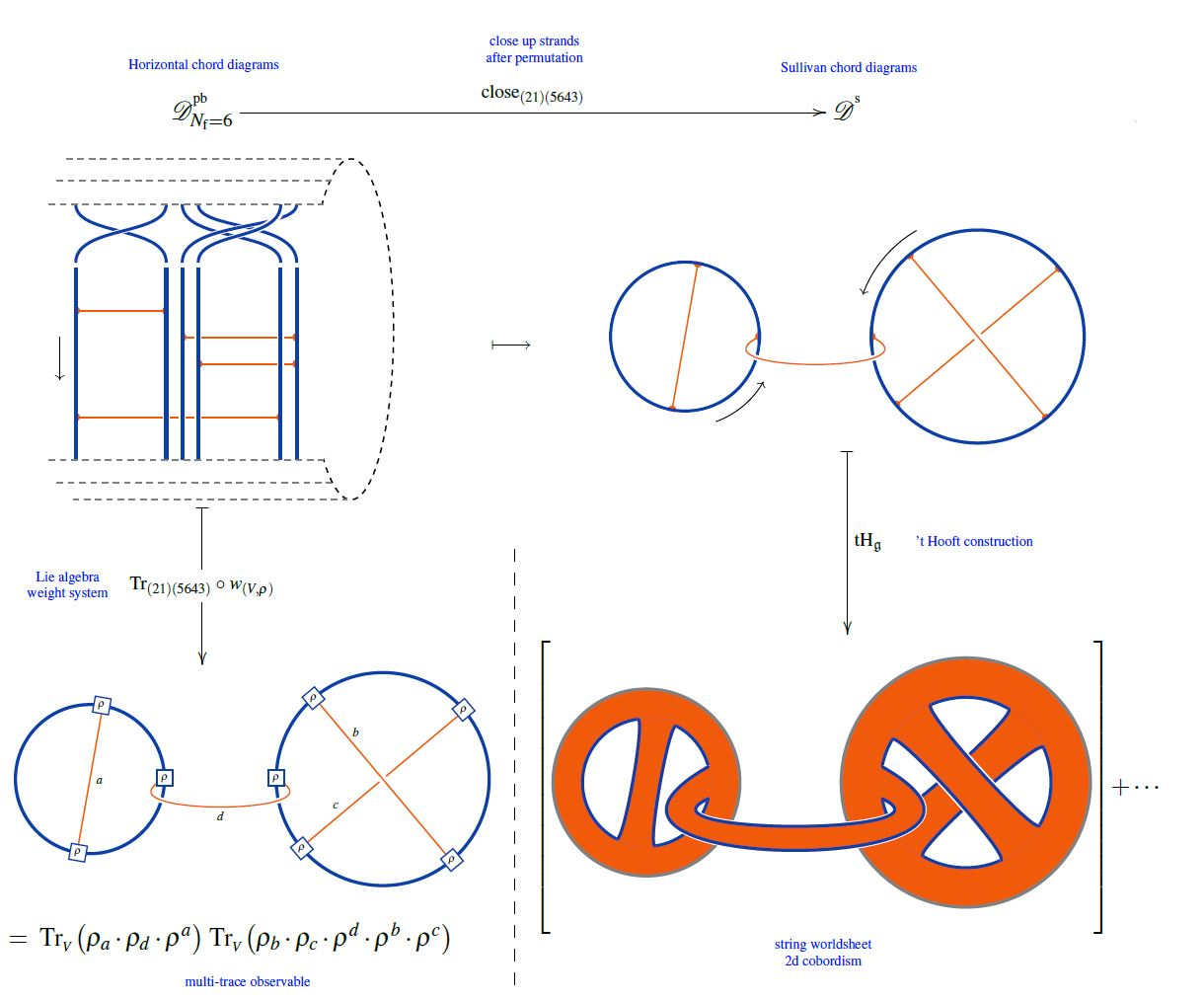(from Sati-Schreiber 19c)

But since all horizontal weight systems are partitioned Lie algebra weight systems this way, this identifies supersymmetric states of the BMN matrix model as seen by invariant multi-trace observables as horizontal chord diagrams evaluated in Lie algebra weight systems.

Original articles:

Textbook accounts:

Discussion of the star-algebra-structure and associated states on horizontal chord diagrams: